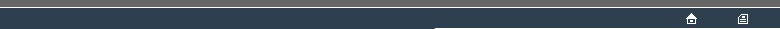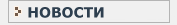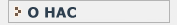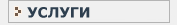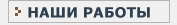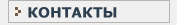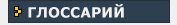СТАТИСТИЧЕСКИЙ В данном разделе Вы найдете все интересующие Вас термины и определения, связанные с статистикой и статистическими исследованиями.КЛИНИЧЕСКИЕ ИССЛЕДОВАНИЯ Необходимый перечень терминов и определений, используемых при проведении клинических испытанийT TAIL An area at the extreme of a RANDOMISATION DISTRIBUTION, where the degree of extremity is sufficient to be notable judged against some NOMINAL ALPHA CRITERION VALUE. Also see : BRANCH-AND BOUND, RE-RANDOMISATION TEST, TAIL DEFINITION POLICY. TAIL DEFINITION POLICY This is a defined method for dividing a DISCRETE DISTRIBUTION into a TAIL area and a body area. The scope for differing policies arises due to the non-infinitesmal amount of probability measure which may be associated with the ACTUAL OUTOME value. The conventional policy, based upon considerations of simplicity and of conservatism in terms of ALPHA, is to include the whole of the weight of outcomes equal to the ACTUAL OUTCOME as part of the TAIL. Also see MID-P, SACROWICZ & COHEN. TEST STATISTIC A STATISTIC measuring the strength of the pattern which a statistical test undertakes to detect. In the context of RE-RANDOMISATION TESTS one is concerned with the distribution of the values of the TEST STATISTIC over the RANDOMISATION SET. An example of a TEST STATISTIC is the DIFFERENCE OF MEANS as employed in the PITMAN PERMUTATION TEST. Also see : EXACT TEST(1), OUTCOME VALUE. TIED RANKS In a NONPARAMETRIC TEST involving RANKED DATA, if two data have TIED VALUES then they will deserve to receive the same rank value. It is generally agreed that this should be the average of the ranks which would have been assigned if the values had been discernably unequal. Thus, the ranks assigned to a set of 6 data, with ties present might emerge as sets such as : 1,3,3,3,5,6 or 1,2,3.5,3.5,5,6. The possibility of TIED RANKS leads to elaborations in the otherwise-standard tasks of computing or tabulating RANDOMISATION DISTRIBUTIONS where data are replaced by ranks. TIED VALUES Where data are represented by ranks, TIED VALUES lead to TIED RANKS. Whether or not data are rep[resnted by ranks, for any TEST STATISTIC the occurrence of TIED VALUES will increase the extent to which a RANDOMISATION DISTRIBUTION will be a DISCRETE DISTRIBUTION rather than a CONTINUOUS DISTRIBUTION. TWO-WAY TABLE A representation of suitable data in a table organised as rows and columns, such that the rows represent one scheme of alternatives covering the whole of the the data represented, the columns represent a further scheme of alternatives covering the whole of the data represented, and the entries in the TWO-WAY TABLE are the counts of numbers of observations conforming to the respective cells of the two-way classification. TYPE-1 ERROR See : ALPHA. TYPE-2 ERROR See : BETA.T
TAIL
TAIL DEFINITION POLICY
TEST STATISTIC
TIED RANKS
TIED VALUES
TWO-WAY TABLE
TYPE-1 ERROR
TYPE-2 ERRORНовости   О нас   Услуги   Наши работы   Статьи   Контакты   Глоссарий
Статистическая помощь! © 2005 - 2023 • Защита авторских прав